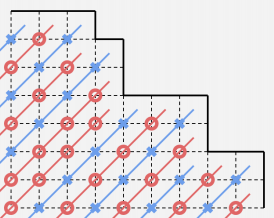AGC002

## D

rose用的是kruskal重构树，时间下界为nlogn，而且是在线的

## E## F

$$f(i,j)=f(i-1,j)+\ f(i,j-1) \times (n-j+1) \times C_{n-i+(k-1)*(n-j+1)-1}^{k-2}$$